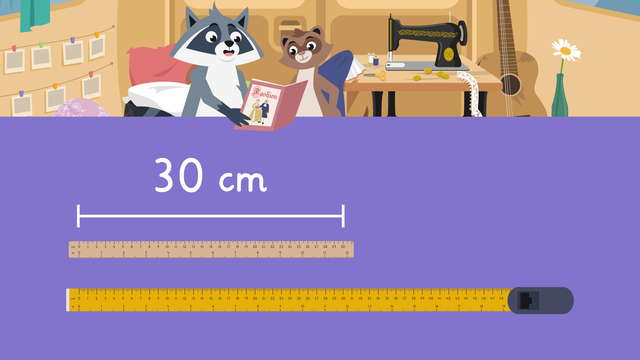# Measuring in CentimetersRate this video

Ø 5.0 / 2 ratings

The authorTeam Digital
Measuring in Centimeters
CCSS.MATH.CONTENT.2.MD.A.1

## Information about the videoMeasuring in Centimeters

### In This Video on Measuring in Centimeters

Zuri and Freddie want to know how to measure in centimeters so they can sew some clothes! They learn all about measuring, and in the end they make some wonderful clothes. Learn what measuring centimeters looks like, and find a measuring centimeter worksheet below.

### Measuring in Centimeters Explained

You can use a centimeter ruler or a centimeter measuring tape to measure objects. The numbers on a ruler when measuring centimeters is the same as centimeter on a measuring tape. The only only difference is that a rule usually ends at 30cm, but tape measures go longer than 30cm!### Measuring in Centimeters Example

To measure using a ruler or a tape measure, first make sure that the object is lined up with the zero on either measuring tool. For example, we line this button up with the zero on the ruler.Then, we find the other end of the object, and find the number that lines up with the far end. For the button, the number two lines up with the end of the object, so the button is two centimeters long.### Centimeter Measuring - Summary

To measure objects using a ruler or measuring tape:

• Line up one end of the object with the zero on your measuring tool
• Find the far end of the object, and find the number that lines up with it
• Record the number, followed by cm to represent centimeters

Below you will find centimeter worksheets - measuring objects.

### TranscriptMeasuring in Centimeters

Zuri and Freddie found an old sewing machine in the landfill today. "Look! There's instructions for sewing clothes, let's make some!" "Sure, but it looks like we need to measure our materials in centimeters, how do we do that?" Let's help Zuri and Freddie learn about measuring in centimeters. A centimeter is a unit of measurement used to measure length. We can measure in centimeters using tools such as a ruler or a tape measure. A ruler is normally used for objects up to thirty centimeters in length. A tape measure can be used for objects up to, and longer than, thirty centimeters. Rulers and tape measures usually have both centimeters and inches. To make sure you use the correct side, look for the side with cm on it. Here is a button Zuri and Freddie need for the clothes. To measure in centimeters, line up one end of the button with the zero mark on the ruler. Now that it is lined up to the zero mark, find the mark that the far end of the button lines up with to find the length. The length of the button is two centimeters, so write a two followed by cm, which represents centimeters. Now we have learned about measuring in centimeters. Let's help Zuri and Freddie measure lace. What is the first step? First, line up one end of the lace with the zero mark on the ruler. What is the next step? Find the mark that the far end of the lace lines up with, to find the length. What is the length of the lace? It is twenty-four centimeters, so write twenty-four followed by cm. Now they know how long the lace is, let's look at the fabric they will use! What is the first step? First, line up one end of the fabric with the zero mark on the ruler. Wait, the fabric is too long for the ruler. What is another tool we could use instead? A tape measure! Line up the end of the fabric with the zero mark on the tape measure this time. What is the next step? Find the mark that the FAR end of the fabric lines up with, to find the length. What is the length of the fabric? It is forty-five centimeters, so write forty-five followed by cm. It looks like Zuri and Freddie know all the measurements of materials they have, so let's review while they sew some clothes! Remember, centimeter is a unit of measurement used to measure length. A ruler or tape measure can help measure in centimeters. To measure in centimeters, first, line up one end of the object with the zero mark on the ruler or tape measure. Second, find the mark that the far end of the object lines up with, to find the length. Finally, record the number followed by cm. "I'm really not too sure about this Freddie..." "Yeah, I have a feeling those sewing instructions are very old..." "I agree... these outfits are... sew-sew."